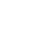## 深入FFM原理与实践

FM和FFM模型是最近几年提出的模型，凭借其在数据量比较大并且特征稀疏的情况下，仍然能够得到优秀的性能和效果的特性，屡次在各大公司举办的CTR预估比赛中获得不错的战绩。美团点评技术团队在搭建DSP的过程中，探索并使用了FM和FFM模型进行CTR和CVR预估，并且取得了不错的效果。本文旨在把我们对FM和FFM原理的探索和应用的经验介绍给有兴趣的读者。

# 前言

1. 首先介绍FM的原理。
2. 其次介绍FFM对FM的改进。
3. 然后介绍FFM的实现细节。
4. 最后介绍模型在DSP场景的应用。

# FM原理

1 USA 26/11/15 Movie
0 China 1/7/14 Game
1 China 19/2/15 Game

1 1 0 1 0 0 1 0
0 0 1 0 1 0 0 1
1 0 1 0 0 1 0 1

One-Hot编码的另一个特点就是导致特征空间大。例如，商品品类有550维特征，一个categorical特征转换为550维数值特征，特征空间剧增。

$y(\mathbf{x}) = w_0+ \sum_{i=1}^n w_i x_i + \sum_{i=1}^n \sum_{j=i+1}^n w_{ij} x_i x_j \label{eq:poly}\tag{1}$$y(\mathbf{x}) = w_0+ \sum_{i=1}^n w_i x_i + \sum_{i=1}^n \sum_{j=i+1}^n \langle \mathbf{v}_i, \mathbf{v}_j \rangle x_i x_j \label{eq:fm}\tag{2}$

$\sum_{i=1}^n \sum_{j=i+1}^n \langle \mathbf{v}_i, \mathbf{v}_j \rangle x_i x_j = \frac{1}{2} \sum_{f=1}^k \left(\left( \sum_{i=1}^n v_{i, f} x_i \right)^2 - \sum_{i=1}^n v_{i, f}^2 x_i^2 \right) \label{eq:fm_conv}\tag{3}$

$\frac{\partial}{\partial\theta} y (\mathbf{x}) = \left\{\begin{array}{ll} 1, & \text{if}\; \theta\; \text{is}\; w_0 \\ x_i, & \text{if}\; \theta\; \text{is}\; w_i \\ x_i \sum_{j=1}^n v_{j, f} x_j - v_{i, f} x_i^2, & \text{if}\; \theta\; \text{is}\; v_{i, f} \end{array}\right.$

## FM与其他模型的对比

FM是一种比较灵活的模型，通过合适的特征变换方式，FM可以模拟二阶多项式核的SVM模型、MF模型、SVD++模型等

# FFM原理

FFM（Field-aware Factorization Machine）最初的概念来自Yu-Chin Juan（阮毓钦，毕业于中国台湾大学，现在美国Criteo工作）与其比赛队员，是他们借鉴了来自Michael Jahrer的论文中的field概念提出了FM的升级版模型。通过引入field的概念，FFM把相同性质的特征归于同一个field。以上面的广告分类为例，“Day=26/11/15”、“Day=1/7/14”、“Day=19/2/15”这三个特征都是代表日期的，可以放到同一个field中。同理，商品的末级品类编码生成了550个特征，这550个特征都是说明商品所属的品类，因此它们也可以放到同一个field中。简单来说，同一个categorical特征经过One-Hot编码生成的数值特征都可以放到同一个field，包括用户性别、职业、品类偏好等。在FFM中，每一维特征 $$x_i$$，针对其它特征的每一种field $$f_j$$，都会学习一个隐向量 $$\mathbf{v}_{i, f_j}$$。因此，隐向量不仅与特征相关，也与field相关。也就是说，“Day=26/11/15”这个特征与“Country”特征和“Ad_type"特征进行关联的时候使用不同的隐向量，这与“Country”和“Ad_type”的内在差异相符，也是FFM中“field-aware”的由来。

$y(\mathbf{x}) = w_0 + \sum_{i=1}^n w_i x_i + \sum_{i=1}^n \sum_{j=i+1}^n \langle \mathbf{v}_{i, f_j}, \mathbf{v}_{j, f_i} \rangle x_i x_j \label{eq:ffm}\tag{4}$

User Movie Genre Price
YuChin 3Idiots Comedy, Drama \$9.99

Field name Field index Feature name Feature index
User 1 User=YuChin 1
Movie 2 Movie=3Idiots 2
Genre 3 Genre=Comedy 3
Price 4 Genre=Drama 4
Price 5

\begin{align*}\begin{array}{r} \langle \mathbf{v}_{{\color{blue}1}, {\color{red}2}}, \mathbf{v}_{{\color{blue}2}, {\color{red}1}} \rangle \cdot {\color{green}1} \cdot {\color{green}1} + \langle \mathbf{v}_{{\color{blue}1}, {\color{red}3}}, \mathbf{v}_{{\color{blue}3}, {\color{red}1}} \rangle \cdot {\color{green}1} \cdot {\color{green}1} + \langle \mathbf{v}_{{\color{blue}1}, {\color{red}3}}, \mathbf{v}_{{\color{blue}4}, {\color{red}1}} \rangle \cdot {\color{green}1} \cdot {\color{green}1} + \langle \mathbf{v}_{{\color{blue}1}, {\color{red}4}}, \mathbf{v}_{{\color{blue}5}, {\color{red}1}} \rangle \cdot {\color{green}1} \cdot {\color{green}{9.99}} \\ {} + \langle \mathbf{v}_{{\color{blue}2}, {\color{red}3}}, \mathbf{v}_{{\color{blue}3}, {\color{red}2}} \rangle \cdot {\color{green}1} \cdot {\color{green}1} + \langle \mathbf{v}_{{\color{blue}2}, {\color{red}3}}, \mathbf{v}_{{\color{blue}4}, {\color{red}2}} \rangle \cdot {\color{green}1} \cdot {\color{green}1} + \langle \mathbf{v}_{{\color{blue}2}, {\color{red}4}}, \mathbf{v}_{{\color{blue}5}, {\color{red}2}} \rangle \cdot {\color{green}1} \cdot {\color{green}{9.99}} \\ {} + \langle \mathbf{v}_{{\color{blue}3}, {\color{red}3}}, \mathbf{v}_{{\color{blue}4}, {\color{red}3}} \rangle \cdot {\color{green}1} \cdot {\color{green}1} + \langle \mathbf{v}_{{\color{blue}3}, {\color{red}4}}, \mathbf{v}_{{\color{blue}5}, {\color{red}3}} \rangle \cdot {\color{green}1} \cdot {\color{green}{9.99}} \\ {} + \langle \mathbf{v}_{{\color{blue}4}, {\color{red}4}}, \mathbf{v}_{{\color{blue}5}, {\color{red}3}} \rangle \cdot {\color{green}1} \cdot {\color{green}{9.99}} \end{array}\end{align*}

# FFM实现

Yu-Chin Juan实现了一个C++版的FFM模型，源码可从Github下载。这个版本的FFM省略了常数项和一次项，模型方程如下。

$\phi(\mathbf{w}, \mathbf{x}) = \sum_{j_1, j_2 \in \mathcal{C}_2} \langle \mathbf{w}_{j_1, f_2}, \mathbf{w}_{j_2, f_1} \rangle x_{j_1} x_{j_2} \label{eq:phi}\tag{5}$

$\min_{\mathbf{w}} \sum_{i=1}^L \log \big( 1 + \exp\{ -y_i \phi (\mathbf{w}, \mathbf{x}_i ) \} \big) + \frac{\lambda}{2} \| \mathbf{w} \|^2$1. 根据样本特征数量（$$tr.n$$）、field的个数（$$tr.m$$）和训练参数（$$pa$$），生成初始化模型，即随机生成模型的参数；
2. 如果归一化参数 $$pa.norm$$ 为真，计算训练和验证样本的归一化系数，样本 $$i$$ 的归一化系数为
$R[i] = \frac{1}{\| \mathbf{X}[i] \|}$
3. 对每一轮迭代，如果随机更新参数 $$pa.rand$$ 为真，随机打乱训练样本的顺序；
4. 对每一个训练样本，执行如下操作
• 计算每一个样本的FFM项，即公式\eqref{eq:phi}中的输出 $$\phi$$；
• 计算每一个样本的训练误差，如算法所示，这里采用的是交叉熵损失函数 $$\log ( 1 + e\phi )$$；
• 利用单个样本的损失函数计算梯度 $$g_\Phi$$，再根据梯度更新模型参数；
5. 对每一个验证样本，计算样本的FFM输出，计算验证误差；
6. 重复步骤3~5，直到迭代结束或验证误差达到最小。

$\mathcal{L} = \mathcal{L}_{err} + \mathcal{L}_{reg} = \log \big( 1 + \exp\{ -y_i \phi(\mathbf{w}, \mathbf{x}_i )\} \big) + \frac{\lambda}{2} \| \mathbf{w} \|^2$

$\frac{\partial\mathcal{L}}{\partial\mathbf{w}} = \frac{\partial\mathcal{L}_{err}}{\partial\phi}\cdot \frac{\partial\phi}{\partial\mathbf{w}} + \frac{\partial\mathcal{L}_{reg}}{\partial\mathbf{w}}$

$w^{'}_{j_1, f_2} = w_{j_1, f_2} - \frac{\eta}{\sqrt{1 + \sum_t (g^t_{w_{j_1, f_2}})^2 }}\cdot g_{w_{j_1, f_2}}$

# FFM应用

CTR和CVR预估模型都是在线下训练，然后用于线上预测。两个模型采用的特征大同小异，主要有三类：用户相关的特征、商品相关的特征、以及用户-商品匹配特征。用户相关的特征包括年龄、性别、职业、兴趣、品类偏好、浏览/购买品类等基本信息，以及用户近期点击量、购买量、消费额等统计信息。商品相关的特征包括所属品类、销量、价格、评分、历史CTR/CVR等信息。用户-商品匹配特征主要有浏览/购买品类匹配、浏览/购买商家匹配、兴趣偏好匹配等几个维度。

CTR、CVR预估样本的类别是按不同方式获取的。CTR预估的正样本是站内点击的用户-商品记录，负样本是展现但未点击的记录；CVR预估的正样本是站内支付（发生转化）的用户-商品记录，负样本是点击但未支付的记录。构建出样本数据后，采用FFM训练预估模型，并测试模型的性能。

#(field) #(feature) AUC Logloss

# 参考文献